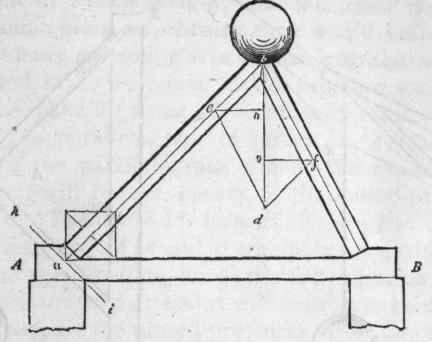In Fig. 24, the weight W presses the struts in the direction of their length; their feet, n n, therefore, tend to move in the direction n 0, and would so move were they not opposed by a sufficient resistance from the blocks, A and A. If a piece of each block be cut off at the horizontal line, a n, the feet of the struts would slide away from each other along that line, in the direction na; but if, instead of these, two pieces were cut off at the vertical line, nb, then the struts would descend vertically. To estimate the horizontal and the vertical pressures exerted by the struts, let n o be made equal (upon any scale of equal parts) to the number of tons with which the strut is pressed; construct the parallelogram of forces by drawing 0 c parallel to an, and of parallel to bn; then nf (by the same scale) shows the number of tons pressure that is exerted by the strut in the direction n a, and n e shows the amount exerted in the direction n b. By constructing designs similar to this, giving various and dissimilar positions to the struts, and then estimating the pressures, it will be found in every case that the horizontal pressure of one strut is exactly equal to that of the other, however much one strut may be inclined more than the other; and also, that the united vertical pressure of the two struts is exactly equal to the weight W. (In this calculation the weight of the timbers has not been taken into consideration, simply to avoid complication to the learner. In practice it is requisite to include the weight of the framing with the load upon the framing.)Fig. 24.

Suppose that the two struts, B and B (Fig, 24), were rafters of a roof, and that instead of the blocks, A and A, the walls of a building were the supports: then, to prevent the walls from being thrown over by the thrust of B and B, it would be desirable to remove the horizontal pressure. This may be done by uniting the feet of the rafters with a rope, iron rod, or piece of timber, as in Fig. 25. This figure is similar to the truss of a roof. The horizontal strains on the tie-beam, tending to pull it asunder in the direction of its length, may be measured at the foot of the rafter, as was shown at Fig. 24; but. it can be more readily and as accurately measured by drawing from f and e horizontal lines to the vertical line, bd, meeting it in 0 and 0; then/0 will be the horizontal thrust at B, and eo at A; these will be found to equal one another. When the rafters of a roof are thus connected, all tendency to thrust out the walls horizontally is removed, the only pressure on them is in a vertical direction, being equal to the weight of the roof and whatever it has to support. This pressure is beneficial rather than otherwise, as a roof having trusses thus formed, and the trusses well braced to each other, tends to steady the walls.Fig. 25.